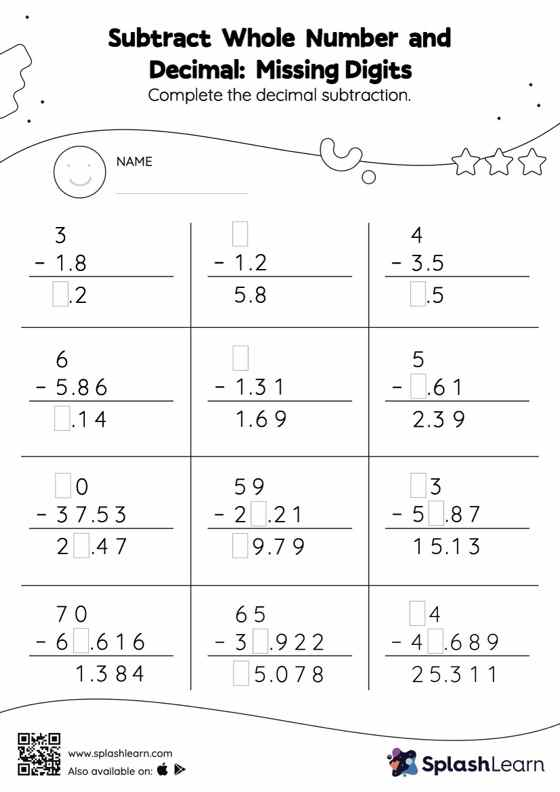# Subtract Whole Number and Decimal: Missing Digits Worksheet

Home > Subtract Whole Number and Decimal: Missing DigitsThis worksheet gives students an opportunity to apply the concepts of subtraction to subtract whole number and decimal. Students align the decimal points and use zero as space holders to subtract decimals. Then they use the relationship between addition and subtraction to find the missing number in subtract whole number and decimal worksheet. This worksheet is about practicing the column method in which numbers are written one on top of another. In this method, students use their place value understanding to solve the problems.